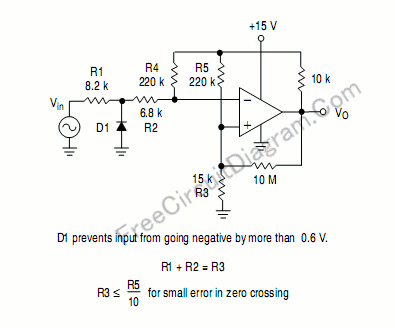# Single Supply Zero Crossing DetectorZero crossing detector is used to convert sine wave or other signal into square-wave, the output should be low if the input is negative and high if the input if positive. Many zero crossing detector use split supply (symmetric supply), but this zero crossing detector circuit only need a single supply, thus suitable for battery-operated circuits. Here is the schematic diagram:The op-amp uses LM339, LM239, LM2901, LM2901V, NCV2901, or MC3302 single supply quad comparator. [Circuit’s schematic diagram source: Motorola Application Notes]